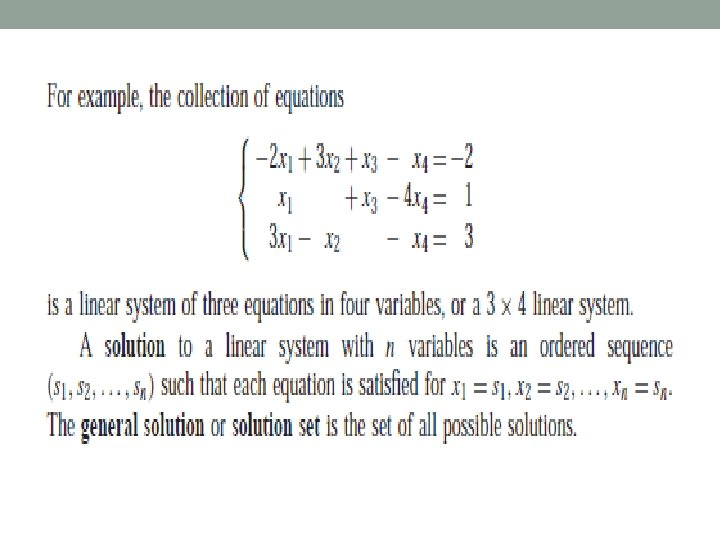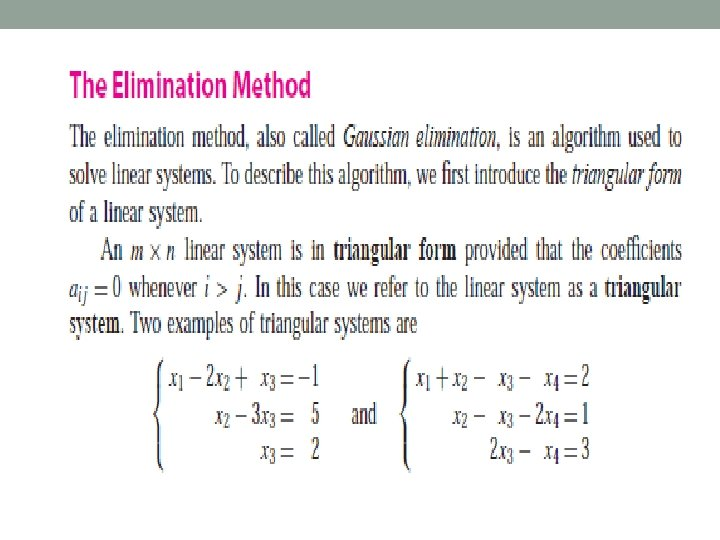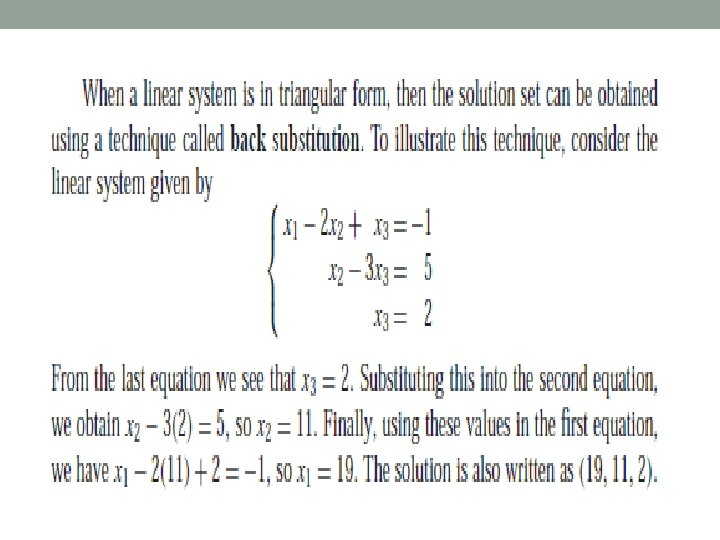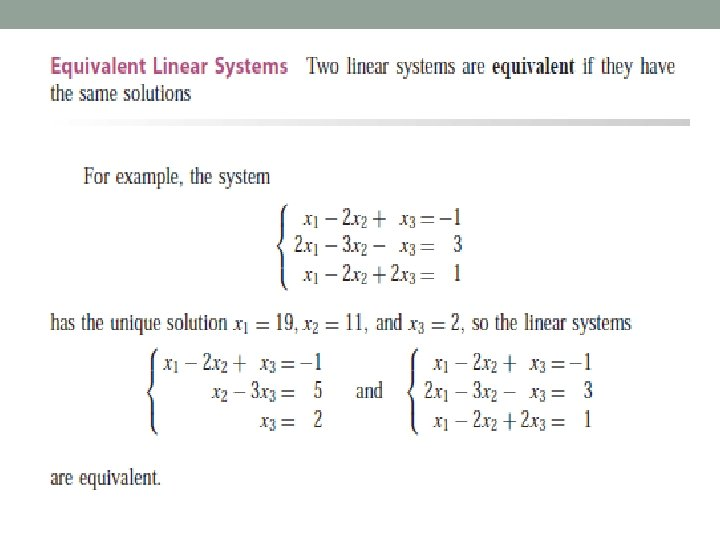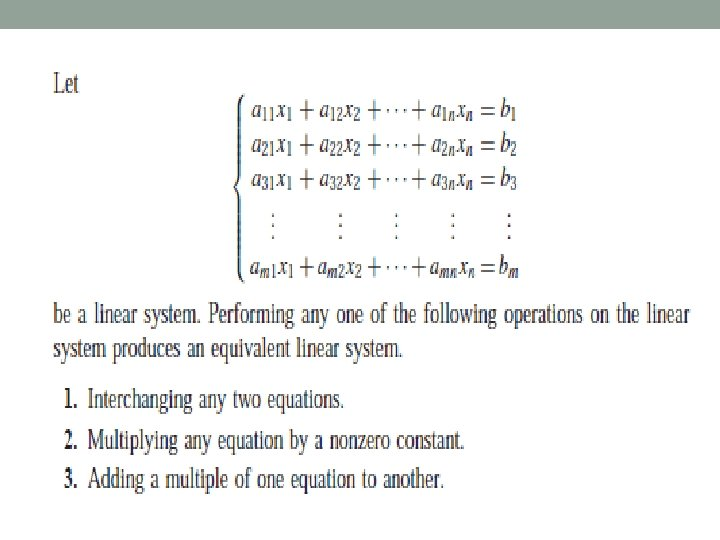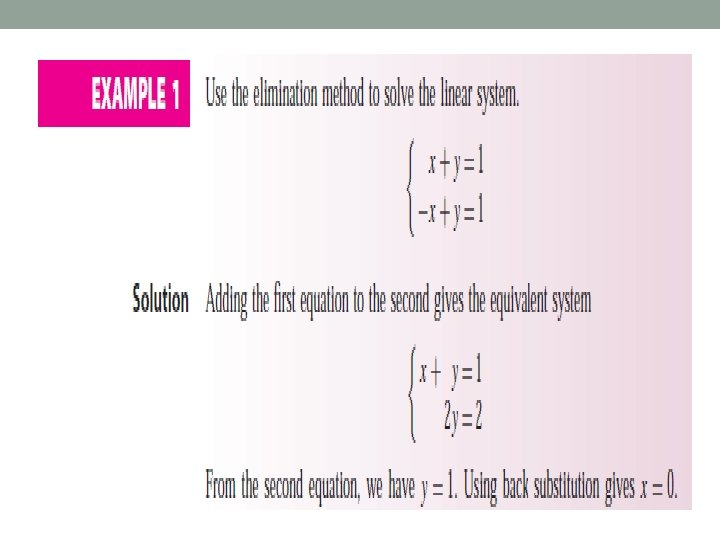# Systems of Linear Equations Systems of Linear Equations

• Slides: 15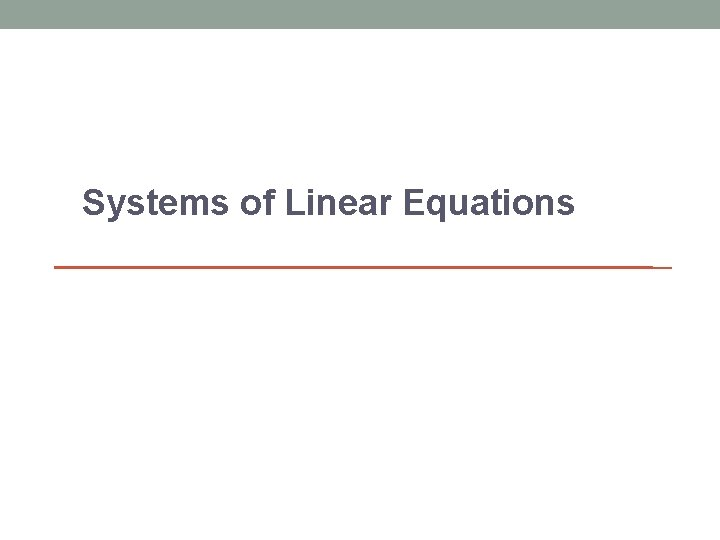Systems of Linear Equations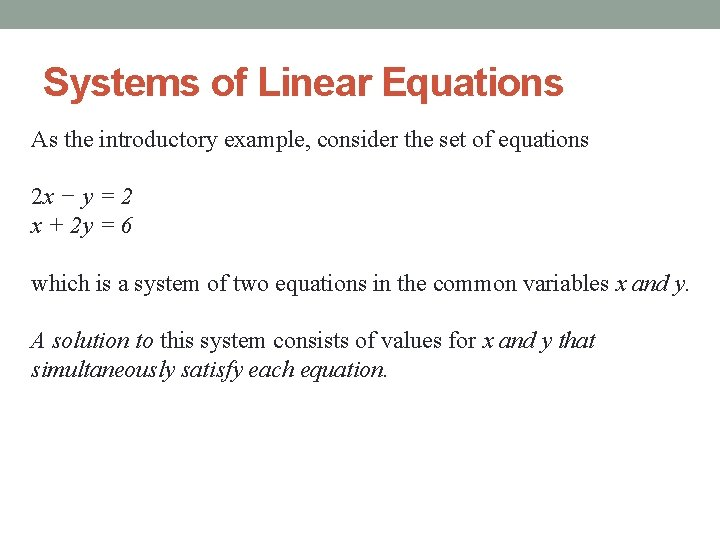Systems of Linear Equations As the introductory example, consider the set of equations 2 x − y = 2 x + 2 y = 6 which is a system of two equations in the common variables x and y. A solution to this system consists of values for x and y that simultaneously satisfy each equation.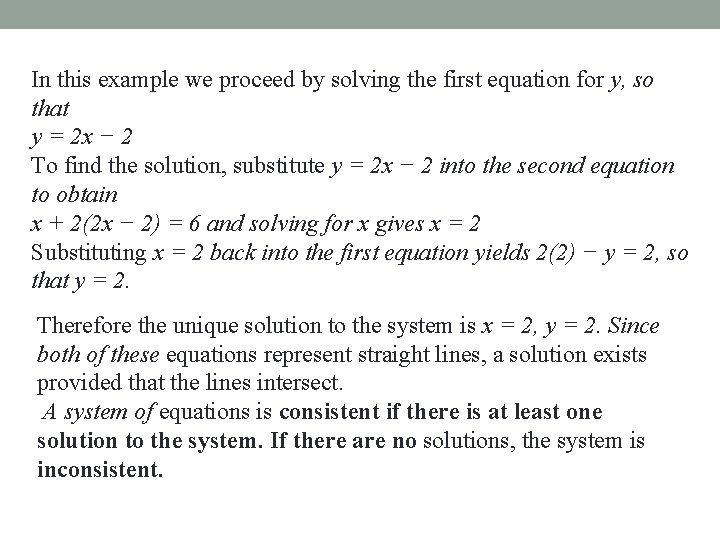In this example we proceed by solving the first equation for y, so that y = 2 x − 2 To find the solution, substitute y = 2 x − 2 into the second equation to obtain x + 2(2 x − 2) = 6 and solving for x gives x = 2 Substituting x = 2 back into the first equation yields 2(2) − y = 2, so that y = 2. Therefore the unique solution to the system is x = 2, y = 2. Since both of these equations represent straight lines, a solution exists provided that the lines intersect. A system of equations is consistent if there is at least one solution to the system. If there are no solutions, the system is inconsistent.In the case of systems of two linear equations with two variables, there are three possibilities: 1. The two lines have different slopes and hence intersect at a unique point, as shown in Fig. 1(a). 2. The two lines are identical (one equation is a nonzero multiple of the other), so there are infinitely many solutions, as shown in Fig. 1(b). 3. The two lines are parallel (have the same slope) and do not intersect, so the system is inconsistent, as shown in Fig. 1(c).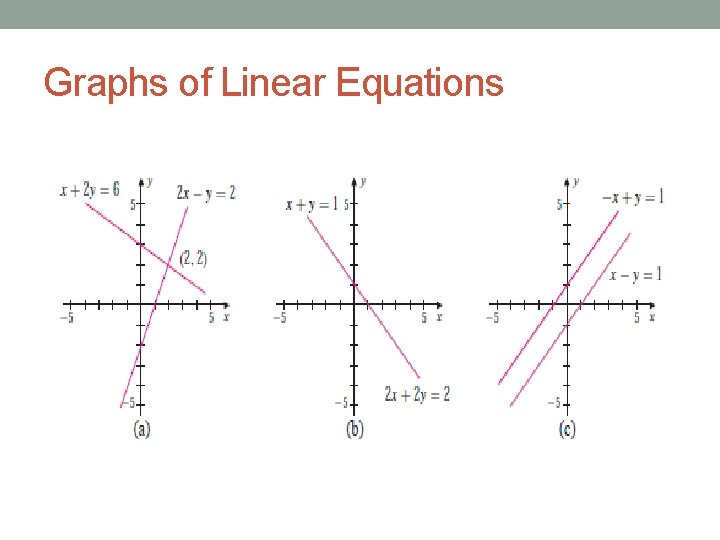Graphs of Linear Equations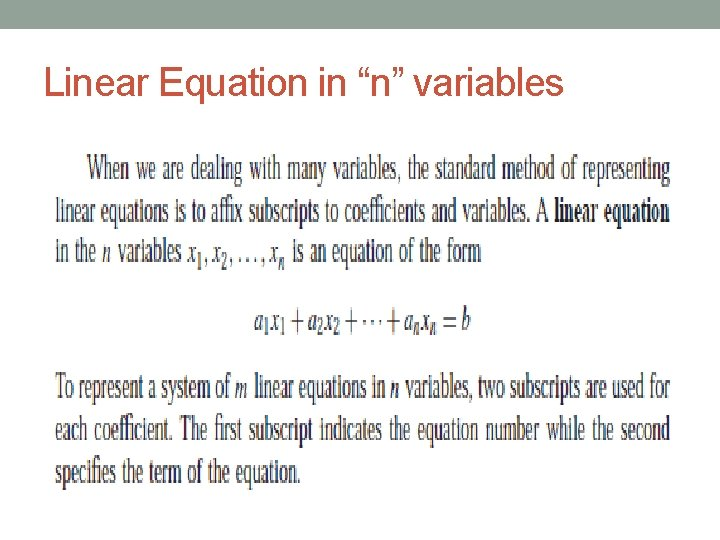Linear Equation in “n” variables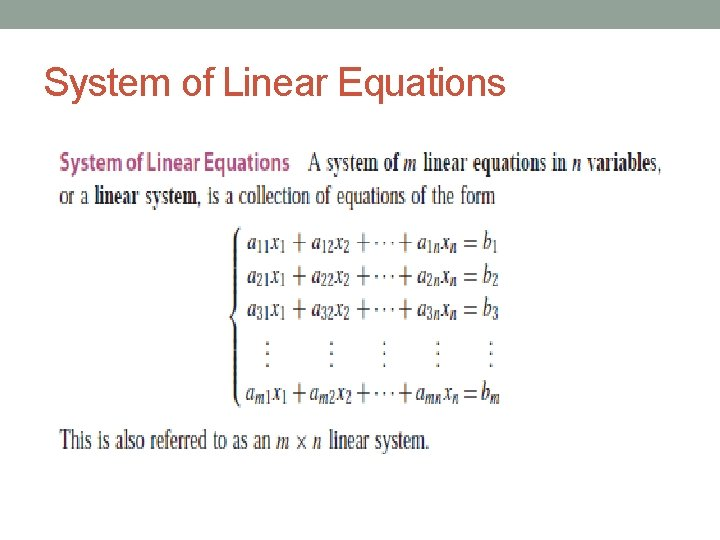System of Linear Equations